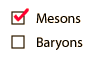Mesons are combinations of two quarks.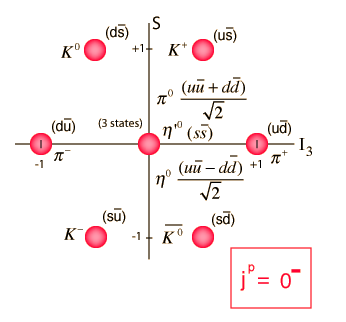The pseudoscalar mesons have quark and antiquark spins antialigned and zero orbital angular momentum, resulting in j=0. They have negative parity. The diagrams are plotted with strangeness on the vertical axis and isospin on the horizontal axis.

 Table of mesons
Index

Particle concepts

 HyperPhysics***** Quantum Physics R Nave
Go BackMesons are combinations of two quarks.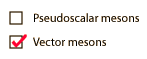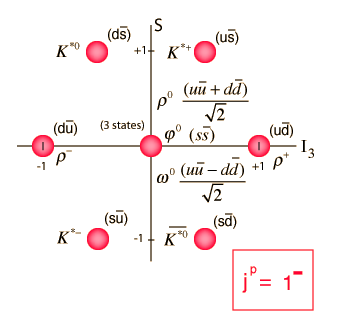The vector mesons have quark and antiquark spins aligned and zero orbital angular momentum, resulting in j=1. They have negative parity. The diagrams are plotted with strangeness on the vertical axis and isospin on the horizontal axis.

The rho vector meson has a mass dramatically larger than the pseudoscalar pi meson which has the same quark content, illustrating the extraordinary variation of effective quark masses.

 Table of mesons
Index

Particle concepts

 HyperPhysics***** Quantum Physics R Nave
Go Back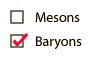Baryons are combinations of three quarks.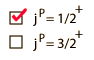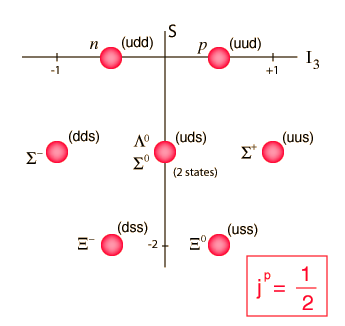The diagrams are plotted with strangeness on the vertical axis and isospin projection on the horizontal axis.

Table of Baryons

Index

Particle concepts

 HyperPhysics***** Quantum Physics R Nave
Go BackBaryons are combinations of three quarks.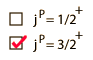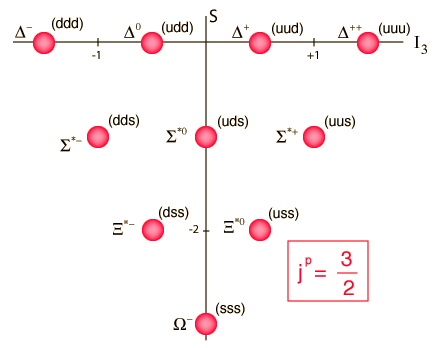The diagrams are plotted with strangeness on the vertical axis and isospin projection on the horizontal axis.

Table of Baryons

Index

Particle concepts

 HyperPhysics***** Quantum Physics R Nave
Go Back